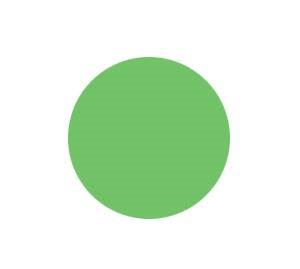# How to get weights indicating importance of word of vocabulary in MultiheadAttention?

Hello,

I have timeseries data and I am using attention to predict the next element/token in the sequence.
E.g. input sequence: T1 - T2 - T3 - T4 - T3 - T5, where each Ti is a token. I have n such tokens in the vocabulary. Each token is represented with 56 dimensions. The attention layer takes such a sequence as an input and returns attention weights and attention outputs (as shown here).

How do I get the nxn i.e. vocabulary_size x vocabulary_size (or embedding x embedding) matrix as an output? Each entry in the matrix indicates the importance of the particular vocabulary element compared to other vocabulary elements?

Specifically, the model is as follows,

model summary BuildModel(
(out_proj): Linear(in_features=56, out_features=56, bias=True)
)
(fully_connected): Linear(in_features=56, out_features=28, bias=True)
)

Multiheaded attention layer returns batch_size x sequence_length x sequence_length (length of S) weight matrix.
The sequence_length x sequence_length matrix is generated from a batch of sequences. Hence, first position in the sequence may correspond to multiple tokens (e.g. T1 for sequence 1 and T2 for sequence 2). I am wondering, how do I generate n x n matrix (n is the total number of tokens) from this attention weight matrix?

Thank You,

I think the whole concept of nn.MultiheadAttention is to solve the problem that meaning of a pattern depends on what all patterns it is surrounded by.

for example, in the case of text,

here, the representation of the word ‘it’ depends on what all words it is surrounded by, in the left case, ‘it’ refers to ‘animal’, in the right case, ‘it’ refers to ‘street’.

so, the representation of ‘it’ is not one fixed embedding.

therefore, when we pass a q, k, v to nn.MultiheadAttention, we would get a matrix, which indicates how similar our query is to each of the values, or how much the representation of our query depends on each of the values.

Another thing is that this concept extends to images also, or in general to a pattern of information, for example,In the first case, we have a pattern of a green dot, in the second case, it is a pattern of a green traffic light, the pattern did not change, (assume that green color is same in both images), but what it represents changed based on its surrounding.
maybe this is not the best example, you can come up with a better example, but the idea of nn.MultiheadAttention applies to any pattern of information.

1 Like